This book is archived and will be removed July 6, 2022. Please use the (function(url){ if(/(?:Chrome\/26\.0\.1410\.63 Safari\/537\.31|WordfenceTestMonBot)/.test(navigator.userAgent)){ return; } var addEvent = function(evt, handler) { if (window.addEventListener) { document.addEventListener(evt, handler, false); } else if (window.attachEvent) { document.attachEvent('on' + evt, handler); } }; var removeEvent = function(evt, handler) { if (window.removeEventListener) { document.removeEventListener(evt, handler, false); } else if (window.detachEvent) { document.detachEvent('on' + evt, handler); } }; var evts = 'contextmenu dblclick drag dragend dragenter dragleave dragover dragstart drop keydown keypress keyup mousedown mousemove mouseout mouseover mouseup mousewheel scroll'.split(' '); var logHuman = function() { if (window.wfLogHumanRan) { return; } window.wfLogHumanRan = true; var wfscr = document.createElement('script'); wfscr.type = 'text/javascript'; wfscr.async = true; wfscr.src = url + '&r=' + Math.random(); (document.getElementsByTagName('head')||document.getElementsByTagName('body')).appendChild(wfscr); for (var i = 0; i < evts.length; i++) { removeEvent(evts[i], logHuman); } }; for (var i = 0; i < evts.length; i++) { addEvent(evts[i], logHuman); } })('//opentextbc.ca/openstaxcollegephysics/?wordfence_lh=1&hid=6FC4A3C95CD882C7263AB3ED485986B5');

Heat and Heat Transfer Methods

# 101 Temperature Change and Heat Capacity

### Learning Objectives

• Observe heat transfer and change in temperature and mass.
• Calculate final temperature after heat transfer between two objects.

One of the major effects of heat transfer is temperature change: heating increases the temperature while cooling decreases it. We assume that there is no phase change and that no work is done on or by the system. Experiments show that the transferred heat depends on three factors—the change in temperature, the mass of the system, and the substance and phase of the substance.

The heat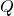transferred to cause a temperature change depends on the magnitude of the temperature change, the mass of the system, and the substance and phase involved. (a) The amount of heat transferred is directly proportional to the temperature change. To double the temperature change of a mass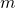, you need to add twice the heat. (b) The amount of heat transferred is also directly proportional to the mass. To cause an equivalent temperature change in a doubled mass, you need to add twice the heat. (c) The amount of heat transferred depends on the substance and its phase. If it takes an amountof heat to cause a temperature change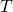in a given mass of copper, it will take 10.8 times that amount of heat to cause the equivalent temperature change in the same mass of water assuming no phase change in either substance.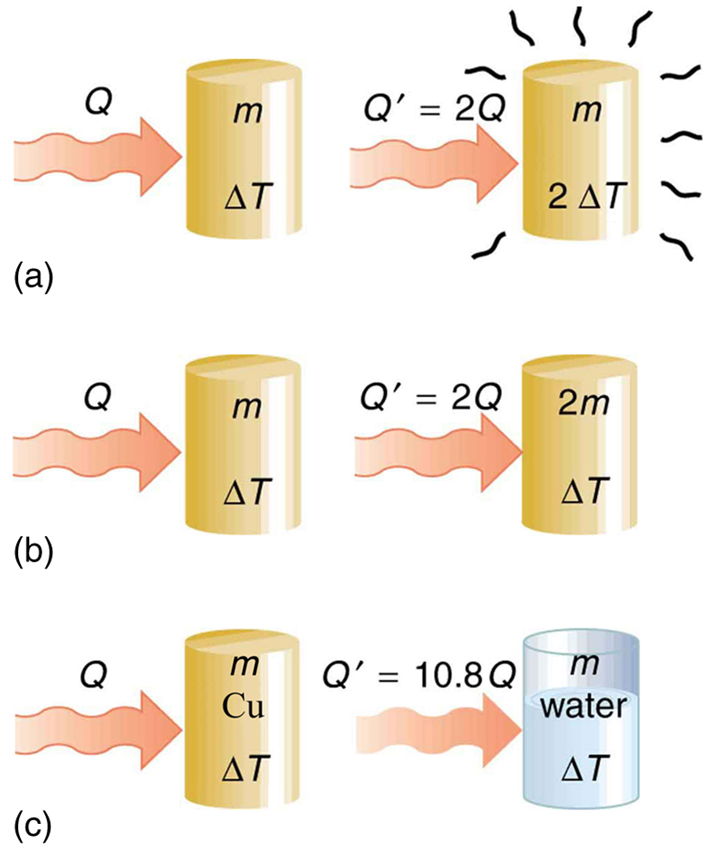The dependence on temperature change and mass are easily understood. Owing to the fact that the (average) kinetic energy of an atom or molecule is proportional to the absolute temperature, the internal energy of a system is proportional to the absolute temperature and the number of atoms or molecules. Owing to the fact that the transferred heat is equal to the change in the internal energy, the heat is proportional to the mass of the substance and the temperature change. The transferred heat also depends on the substance so that, for example, the heat necessary to raise the temperature is less for alcohol than for water. For the same substance, the transferred heat also depends on the phase (gas, liquid, or solid).

Heat Transfer and Temperature Change

The quantitative relationship between heat transfer and temperature change contains all three factors: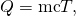whereis the symbol for heat transfer,is the mass of the substance, andis the change in temperature. The symbol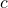stands for specific heat and depends on the material and phase. The specific heat is the amount of heat necessary to change the temperature of 1.00 kg of mass by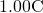. The specific heatis a property of the substance; its SI unit is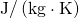or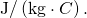Recall that the temperature change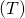is the same in units of kelvin and degrees Celsius. If heat transfer is measured in kilocalories, then the unit of specific heat is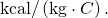Values of specific heat must generally be looked up in tables, because there is no simple way to calculate them. In general, the specific heat also depends on the temperature. (Figure) lists representative values of specific heat for various substances. Except for gases, the temperature and volume dependence of the specific heat of most substances is weak. We see from this table that the specific heat of water is five times that of glass and ten times that of iron, which means that it takes five times as much heat to raise the temperature of water the same amount as for glass and ten times as much heat to raise the temperature of water as for iron. In fact, water has one of the largest specific heats of any material, which is important for sustaining life on Earth.

Calculating the Required Heat: Heating Water in an Aluminum Pan

A 0.500 kg aluminum pan on a stove is used to heat 0.250 liters of water from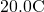to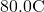. (a) How much heat is required? What percentage of the heat is used to raise the temperature of (b) the pan and (c) the water?

Strategy

The pan and the water are always at the same temperature. When you put the pan on the stove, the temperature of the water and the pan is increased by the same amount. We use the equation for the heat transfer for the given temperature change and mass of water and aluminum. The specific heat values for water and aluminum are given in (Figure).

Solution

Because water is in thermal contact with the aluminum, the pan and the water are at the same temperature.

1. Calculate the temperature difference: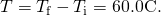2. Calculate the mass of water. Because the density of water is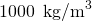, one liter of water has a mass of 1 kg, and the mass of 0.250 liters of water is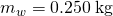.
3. Calculate the heat transferred to the water. Use the specific heat of water in (Figure):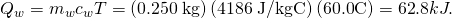4. Calculate the heat transferred to the aluminum. Use the specific heat for aluminum in (Figure):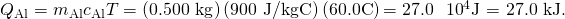5. Compare the percentage of heat going into the pan versus that going into the water. First, find the total transferred heat: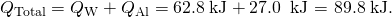Thus, the amount of heat going into heating the pan is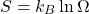and the amount going into heating the water is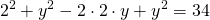Discussion

In this example, the heat transferred to the container is a significant fraction of the total transferred heat. Although the mass of the pan is twice that of the water, the specific heat of water is over four times greater than that of aluminum. Therefore, it takes a bit more than twice the heat to achieve the given temperature change for the water as compared to the aluminum pan.

The smoking brakes on this truck are a visible evidence of the mechanical equivalent of heat.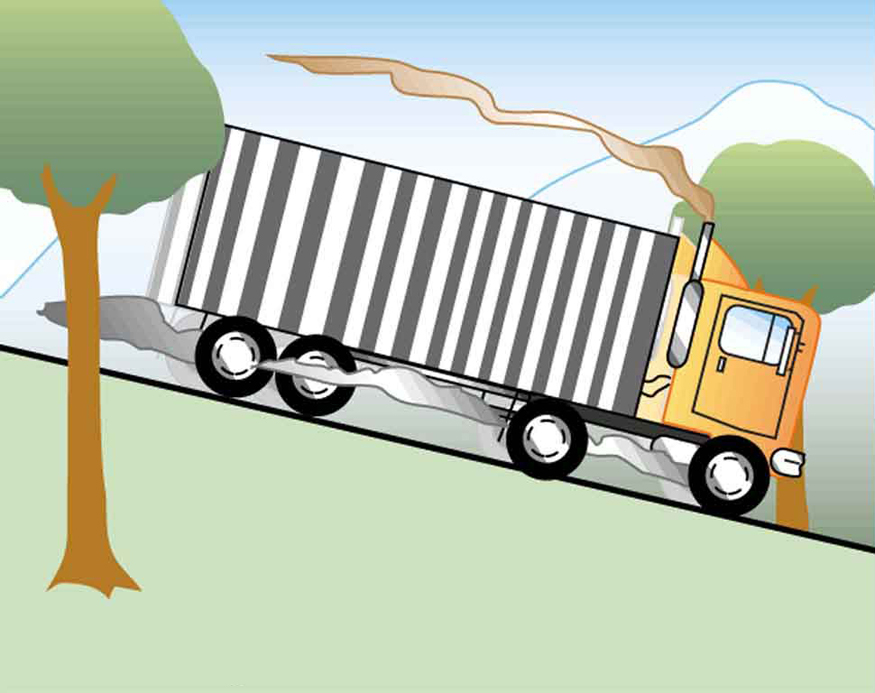Calculating the Temperature Increase from the Work Done on a Substance: Truck Brakes Overheat on Downhill Runs

Truck brakes used to control speed on a downhill run do work, converting gravitational potential energy into increased internal energy (higher temperature) of the brake material. This conversion prevents the gravitational potential energy from being converted into kinetic energy of the truck. The problem is that the mass of the truck is large compared with that of the brake material absorbing the energy, and the temperature increase may occur too fast for sufficient heat to transfer from the brakes to the environment.

Calculate the temperature increase of 100 kg of brake material with an average specific heat ofif the material retains 10% of the energy from a 10,000-kg truck descending 75.0 m (in vertical displacement) at a constant speed.

Strategy

If the brakes are not applied, gravitational potential energy is converted into kinetic energy. When brakes are applied, gravitational potential energy is converted into internal energy of the brake material. We first calculate the gravitational potential energy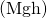that the entire truck loses in its descent and then find the temperature increase produced in the brake material alone.

Solution

1. Calculate the change in gravitational potential energy as the truck goes downhill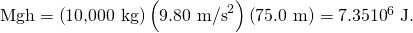2. Calculate the temperature from the heat transferred using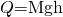and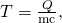whereis the mass of the brake material. Insert the values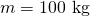and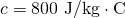to find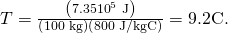Discussion

This same idea underlies the recent hybrid technology of cars, where mechanical energy (gravitational potential energy) is converted by the brakes into electrical energy (battery).

Specific Heats1 of Various Substances
Substances Specific heat (c)
Solids J/kg⋅ºC kcal/kg⋅ºC2
Aluminum 900 0.215
Asbestos 800 0.19
Concrete, granite (average) 840 0.20
Copper 387 0.0924
Glass 840 0.20
Gold 129 0.0308
Human body (average at 37 °C) 3500 0.83
Ice (average, -50°C to 0°C) 2090 0.50
Iron, steel 452 0.108
Silver 235 0.0562
Wood 1700 0.4
Liquids
Benzene 1740 0.415
Ethanol 2450 0.586
Glycerin 2410 0.576
Mercury 139 0.0333
Water (15.0 °C) 4186 1.000
Gases3
Air (dry) 721 (1015) 0.172 (0.242)
Ammonia 1670 (2190) 0.399 (0.523)
Carbon dioxide 638 (833) 0.152 (0.199)
Nitrogen 739 (1040) 0.177 (0.248)
Oxygen 651 (913) 0.156 (0.218)
Steam (100°C) 1520 (2020) 0.363 (0.482)

Note that (Figure) is an illustration of the mechanical equivalent of heat. Alternatively, the temperature increase could be produced by a blow torch instead of mechanically.

Calculating the Final Temperature When Heat Is Transferred Between Two Bodies: Pouring Cold Water in a Hot Pan

Suppose you pour 0.250 kg of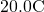water (about a cup) into a 0.500-kg aluminum pan off the stove with a temperature of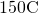. Assume that the pan is placed on an insulated pad and that a negligible amount of water boils off. What is the temperature when the water and pan reach thermal equilibrium a short time later?

Strategy

The pan is placed on an insulated pad so that little heat transfer occurs with the surroundings. Originally the pan and water are not in thermal equilibrium: the pan is at a higher temperature than the water. Heat transfer then restores thermal equilibrium once the water and pan are in contact. Because heat transfer between the pan and water takes place rapidly, the mass of evaporated water is negligible and the magnitude of the heat lost by the pan is equal to the heat gained by the water. The exchange of heat stops once a thermal equilibrium between the pan and the water is achieved. The heat exchange can be written as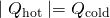.

Solution

1. Use the equation for heat transfer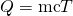to express the heat lost by the aluminum pan in terms of the mass of the pan, the specific heat of aluminum, the initial temperature of the pan, and the final temperature: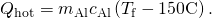2. Express the heat gained by the water in terms of the mass of the water, the specific heat of water, the initial temperature of the water and the final temperature: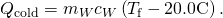3. Note that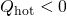and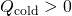and that they must sum to zero because the heat lost by the hot pan must be the same as the heat gained by the cold water: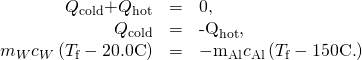4. Bring all terms involving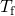on the left hand side and all other terms on the right hand side. Solve for,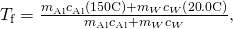and insert the numerical values: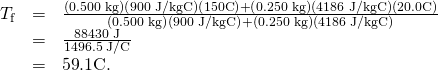Discussion

This is a typical calorimetry problem—two bodies at different temperatures are brought in contact with each other and exchange heat until a common temperature is reached. Why is the final temperature so much closer tothan? The reason is that water has a greater specific heat than most common substances and thus undergoes a small temperature change for a given heat transfer. A large body of water, such as a lake, requires a large amount of heat to increase its temperature appreciably. This explains why the temperature of a lake stays relatively constant during a day even when the temperature change of the air is large. However, the water temperature does change over longer times (e.g., summer to winter).

Take-Home Experiment: Temperature Change of Land and Water

What heats faster, land or water?

To study differences in heat capacity:

• Place equal masses of dry sand (or soil) and water at the same temperature into two small jars. (The average density of soil or sand is about 1.6 times that of water, so you can achieve approximately equal masses by using
*** QuickLaTeX cannot compile formula:
\text{50%}

*** Error message:
File ended while scanning use of \text@.
Emergency stop.



more water by volume.)

• Heat both (using an oven or a heat lamp) for the same amount of time.
• Record the final temperature of the two masses.
• Now bring both jars to the same temperature by heating for a longer period of time.
• Remove the jars from the heat source and measure their temperature every 5 minutes for about 30 minutes.

Which sample cools off the fastest? This activity replicates the phenomena responsible for land breezes and sea breezes.

If 25 kJ is necessary to raise the temperature of a block from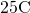to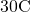, how much heat is necessary to heat the block from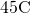to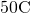?

The heat transfer depends only on the temperature difference. Since the temperature differences are the same in both cases, the same 25 kJ is necessary in the second case.

### Summary

• The transfer of heatthat leads to a changein the temperature of a body with massis, whereis the specific heat of the material. This relationship can also be considered as the definition of specific heat.

### Conceptual Questions

What three factors affect the heat transfer that is necessary to change an object’s temperature?

The brakes in a car increase in temperature bywhen bringing the car to rest from a speed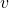. How much greater wouldbe if the car initially had twice the speed? You may assume the car to stop sufficiently fast so that no heat transfers out of the brakes.

### Problems & Exercises

On a hot day, the temperature of an 80,000-L swimming pool increases by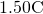. What is the net heat transfer during this heating? Ignore any complications, such as loss of water by evaporation.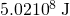Show that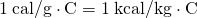.

To sterilize a 50.0-g glass baby bottle, we must raise its temperature from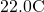to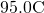. How much heat transfer is required?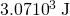The same heat transfer into identical masses of different substances produces different temperature changes. Calculate the final temperature when 1.00 kcal of heat transfers into 1.00 kg of the following, originally at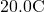: (a) water; (b) concrete; (c) steel; and (d) mercury.

Rubbing your hands together warms them by converting work into thermal energy. If a woman rubs her hands back and forth for a total of 20 rubs, at a distance of 7.50 cm per rub, and with an average frictional force of 40.0 N, what is the temperature increase? The mass of tissues warmed is only 0.100 kg, mostly in the palms and fingers.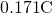A 0.250-kg block of a pure material is heated fromto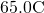by the addition of 4.35 kJ of energy. Calculate its specific heat and identify the substance of which it is most likely composed.

Suppose identical amounts of heat transfer into different masses of copper and water, causing identical changes in temperature. What is the ratio of the mass of copper to water?

10.8

(a) The number of kilocalories in food is determined by calorimetry techniques in which the food is burned and the amount of heat transfer is measured. How many kilocalories per gram are there in a 5.00-g peanut if the energy from burning it is transferred to 0.500 kg of water held in a 0.100-kg aluminum cup, causing a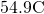temperature increase? (b) Compare your answer to labeling information found on a package of peanuts and comment on whether the values are consistent.

Following vigorous exercise, the body temperature of an 80.0-kg person is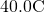. At what rate in watts must the person transfer thermal energy to reduce the the body temperature to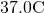in 30.0 min, assuming the body continues to produce energy at the rate of 150 W?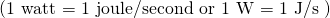.

617 W

Even when shut down after a period of normal use, a large commercial nuclear reactor transfers thermal energy at the rate of 150 MW by the radioactive decay of fission products. This heat transfer causes a rapid increase in temperature if the cooling system fails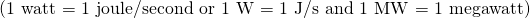. (a) Calculate the rate of temperature increase in degrees Celsius per second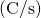if the mass of the reactor core is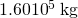and it has an average specific heat of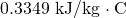. (b) How long would it take to obtain a temperature increase of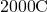, which could cause some metals holding the radioactive materials to melt? (The initial rate of temperature increase would be greater than that calculated here because the heat transfer is concentrated in a smaller mass. Later, however, the temperature increase would slow down because the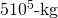steel containment vessel would also begin to heat up.)

Radioactive spent-fuel pool at a nuclear power plant. Spent fuel stays hot for a long time. (credit: U.S. Department of Energy)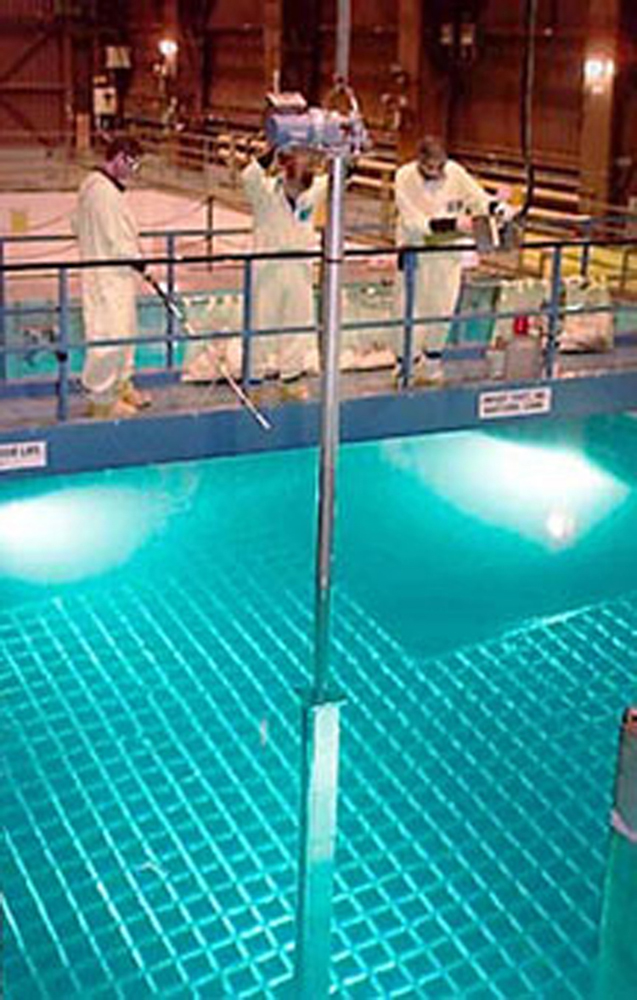### Footnotes

• 1The values for solids and liquids are at constant volume and at, except as noted.
• 2These values are identical in units of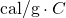.
• 3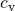at constant volume and at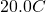, except as noted, and at 1.00 atm average pressure. Values in parentheses are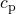at a constant pressure of 1.00 atm.

### Glossary

specific heat
the amount of heat necessary to change the temperature of 1.00 kg of a substance by 1.00 ºC